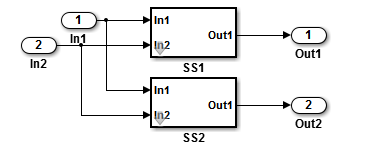Main Content

## Determine Why Subsystem Code Is Not Reused

Due to the limitations described in Generate Reentrant Code from Subsystems, the code generator might not reuse generated code as you expect. To determine why code generated for a subsystem is not reused:

1. Review the Subsystems section of the code generation report.

2. Compare subsystem checksum data.

### Review Subsystems Section of HTML Code Generation Report

If the code generator does not generate code for a subsystem as reusable code, and you configured the subsystem as reusable, examine the Subsystems section of the code generation report (see Generate a Code Generation Report). The Subsystems section contains:

• A table that summarizes how nonvirtual subsystems were converted to generated code.

• Diagnostic information that describes why subsystems were not generated as reusable code.

The Subsystems section also maps noninlined subsystems in the model to functions or reused functions in the generated code. For an example, open and build the `rtwdemo_atomic` model.

### Compare Subsystem Checksum Data

You can determine why subsystem code is not reused by comparing subsystem checksum data. The code generator determines whether subsystems are identical by comparing subsystem checksums, as noted in Limitations. For subsystem reuse across referenced models, this procedure might not flag every difference.

Consider the model, `rtwdemo_ssreuse`. `SS1` and `SS2` are instances of the same subsystem. In both instances the subsystem block parameter Function packaging is set to ```Reusable function```.Use the method `Simulink.SubSystem.getChecksum` to get the checksum for a subsystem. Review the results to determine why code is not reused.

1. Open the model `rtwdemo_ssreuse`. Save a copy of the model in a folder where you have write access.

2. Associate the subsystems `SS1` and `SS2` with `gcb`. For each of the subsystems , in the model window, select the subsystem. While the subsystem is selected, in the Command Window, enter:

`SS1 = gcb;`
`SS2 = gcb;`
3. Use the method `Simulink.SubSystem.getChecksum` to get the checksum for each subsystem. This method returns two output values: the checksum value and details on the input used to compute the checksum.

```[chksum1, chksum1_details] = ... Simulink.SubSystem.getChecksum(SS1); [chksum2, chksum2_details] = ... Simulink.SubSystem.getChecksum(SS2);```
4. Compare the two checksum values. The values should be equal based on the subsystem configurations.

```isequal(chksum1, chksum2) ans = 1```
5. To use `Simulink.SubSystem.getChecksum` to determine why the checksums of two subsystems differ, change the data type mode of the output port of SS1 so that it differs from that of SS2.

1. Look under the mask of `SS1`. Right-click the subsystem. In the context menu, select Mask > Look Under Mask.

2. In the block diagram of the subsystem, double-click the Lookup Table block to open the Subsystem Parameters dialog box.

3. Click Data Types.

4. Select block parameter Saturate on integer overflow and click .

6. Get the checksum for `SS1`. Compare the checksums for the two subsystems. This time, the checksums are not equal.

```[chksum1, chksum1_details] = ... Simulink.SubSystem.getChecksum(SS1); isequal(chksum1, chksum2) ans = 0```
7. After you determine that the checksums are different, find out why. The Simulink® engine uses information, such as signal data types, some block parameter values, and block connectivity information, to compute the checksums. To determine why checksums are different, compare the data that computes the checksum values. You can get this information from the second value returned by `Simulink.SubSystem.getChecksum`, which is a structure array with four fields.

Look at the structure `chksum1_details`.

```chksum1_details chksum1_details = ContentsChecksum: [1x1 struct] InterfaceChecksum: [1x1 struct] ContentsChecksumItems: [287x1 struct] InterfaceChecksumItems: [53x1 struct]```

`ContentsChecksum` and `InterfaceChecksum` are component checksums of the subsystem checksum. The remaining two fields, `ContentsChecksumItems` and `InterfaceChecksumItems`, contain the checksum details.

8. Determine whether a difference exists in the subsystem contents, interface, or both. For example:

```isequal(chksum1_details.ContentsChecksum.Value,... chksum2_details.ContentsChecksum.Value) ans = 0 isequal(chksum1_details.InterfaceChecksum.Value,... chksum2_details.InterfaceChecksum.Value) ans = 1```

In this case, differences exist in the content.

9. Write a script like this script to find the differences.

```idxForCDiffs=[]; for idx = 1:length(chksum1_details.ContentsChecksumItems) if (~strcmp(chksum1_details.ContentsChecksumItems(idx).Identifier, ... chksum2_details.ContentsChecksumItems(idx).Identifier)) disp(['Identifiers different for contents item ', num2str(idx)]); idxForCDiffs=[idxForCDiffs, idx]; end if (ischar(chksum1_details.ContentsChecksumItems(idx).Value)) if (~strcmp(chksum1_details.ContentsChecksumItems(idx).Value, ... chksum2_details.ContentsChecksumItems(idx).Value)) disp(['Character vector values different for contents item ', num2str(idx)]); idxForCDiffs=[idxForCDiffs, idx]; end end if (isnumeric(chksum1_details.ContentsChecksumItems(idx).Value)) if (chksum1_details.ContentsChecksumItems(idx).Value ~= ... chksum2_details.ContentsChecksumItems(idx).Value) disp(['Numeric values different for contents item ', num2str(idx)]); idxForCDiffs=[idxForCDiffs, idx]; end end end ```
10. Run the script. The example assumes that you named the script `check_details`.

```check_details Character vector values different for contents item 202```

The results indicate that differences exist for index item 202 in the subsystem contents.

11. Use the returned index values to get the handle, identifier, and value details for each difference found.

```chksum1_details.ContentsChecksumItems(202) ans = Handle: 'rtwdemo_ssreuse/SS1/Lookup Table' Identifier: 'SaturateOnIntegerOverflow' Value: 'on' ```

The details identify the Lookup Table block parameter Saturate on integer overflow as the focus for debugging a subsystem reuse issue.

Download guide